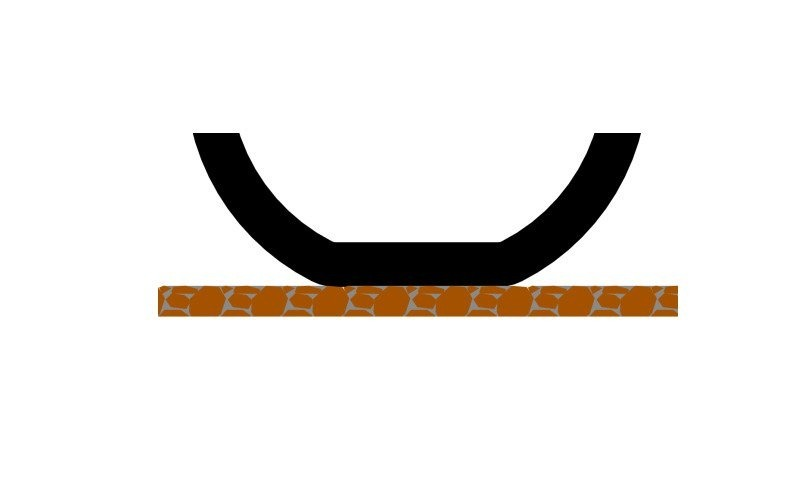The Formula for Friction

f = µ×n

• f = Friction
• µ = Mu or Coefficient of friction (Cf)
• n = Normal down force of weight or mass.

Notice, the size of the surface area is not in this formula.

Coefficient of friction is generated by the two materials rubbing together such as the tire & the pavement. This value could be anywhere from 0.10 to 1.80. A good race tire may develop in the range of 1.60g.

For example, a tire with a Cf. of 1.60g, and 100% of the weight of bike and rider is on the rear tire, then 1.60g acceleration can be achieved, assuming there is enough horsepower available. On the other hand, if only 50% of the bike’s weight is on the rear tire then only 0.80g acceleration is posable.

If you wanted to use surface area in a friction calculation, you would replace N with PSI. The calculation would look like,

f = µ×weight/sq.in. (PSI)

If you simply, increase the size of the contact patch, but keep the same weight, the result is a lower PSI.

For example, using round numbers, if you have one hundred pounds on one square inch of rubber then you have 100psi×1sq.in. If you take the same one hundred pounds and put it on two square inch of rubber then you have only 50psi×2sq.in.

So, in conclusion increasing the surface area of a tire in and of itself does not increase the friction level.

On the other hand, the size of the surface area will come into play when you start to calculate in mechanical stresses on the contact patch. If the surface area is too small for the mass or power of the bike, the tire will mechanically tear and shred resulting in premature and excessive tire spin or slide. If the contact area is too big then the compound will not be able to come to the correct operating temperature and not develop the max coefficient of friction and result in premature and excessive tire spin or slide. Ask your professional tire rep for advice, on the proper size and type of tire for your bike.